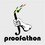# Polynomial Game - Problem 4

Consider a polynomial $f(x)=x^{2014}+a_{2013}x^{2013}+\dots+a_1x+a_0$. Cody and Pi Han take turns choosing one of the coefficients $a_0,a_1,\dots,a_{2013}$ and assigning a real value to it. Once a value is assigned to a coefficient, it cannot be changed anymore. The game ends after all of the coefficients have been assigned values. Pi Han's goal is to make $f(x)$ divisible by a fixed polynomial $m(x)$, and Cody's goal is to prevent this.

• Which of the players has a strategy if $m(x)=x^2+x+1$ and Cody goes first?
• Which of the players has a strategy if $m(x)=x^4-1$ and Cody goes first?Note by Cody Johnson
7 years, 3 months ago

This discussion board is a place to discuss our Daily Challenges and the math and science related to those challenges. Explanations are more than just a solution — they should explain the steps and thinking strategies that you used to obtain the solution. Comments should further the discussion of math and science.

When posting on Brilliant:

• Use the emojis to react to an explanation, whether you're congratulating a job well done , or just really confused .
• Ask specific questions about the challenge or the steps in somebody's explanation. Well-posed questions can add a lot to the discussion, but posting "I don't understand!" doesn't help anyone.
• Try to contribute something new to the discussion, whether it is an extension, generalization or other idea related to the challenge.

MarkdownAppears as
*italics* or _italics_ italics
**bold** or __bold__ bold
- bulleted- list
• bulleted
• list
1. numbered2. list
1. numbered
2. list
Note: you must add a full line of space before and after lists for them to show up correctly
paragraph 1paragraph 2

paragraph 1

paragraph 2

[example link](https://brilliant.org)example link
> This is a quote
This is a quote
    # I indented these lines
# 4 spaces, and now they show
# up as a code block.

print "hello world"
# I indented these lines
# 4 spaces, and now they show
# up as a code block.

print "hello world"
MathAppears as
Remember to wrap math in $$ ... $$ or $ ... $ to ensure proper formatting.
2 \times 3 $2 \times 3$
2^{34} $2^{34}$
a_{i-1} $a_{i-1}$
\frac{2}{3} $\frac{2}{3}$
\sqrt{2} $\sqrt{2}$
\sum_{i=1}^3 $\sum_{i=1}^3$
\sin \theta $\sin \theta$
\boxed{123} $\boxed{123}$

Sort by:

Hardest question in the entire contest! :'(

- 7 years, 3 months ago

Hint: look at the roots of $m(x)$.

- 7 years, 3 months ago

I felt that remainder-factor theorem was a recurring theme in the questions about polynomials.

Staff - 7 years, 3 months ago

By considering unassigned coefficients as they were zero, the polynomial $f(x)$ changes move-by-move. For the first point, Pi Han's goal is to have $f(\omega)=0$, with $\omega$ being a third root of unity, with its last move. Cody can prevent this by playing in such a way that, just after his turns, $\arg f(\omega)$ is not a multiple of $\pi/3$. For the second point, if Cody plays in such a way that, just after his turns, $\arg f(i)$ is not a multiple of $\pi/4$, Pi Han's doomed again, since $f(i)=0$ is a necessary condition in order to have $(x^4-1)\mid f(x)$.

- 7 years, 3 months ago

But Cody goes first.

- 7 years, 3 months ago